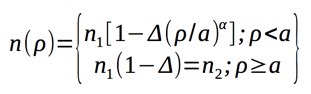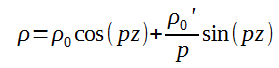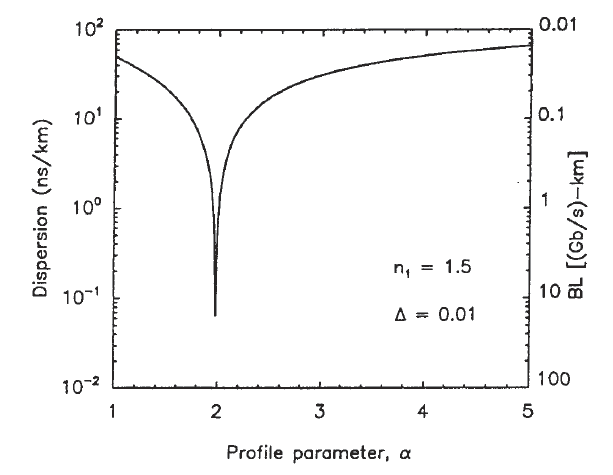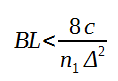The refractive index of the core in graded-index fibers is not constant but decreases gradually from its maximum value n1 at the core center to its minimum value n2 at the core-cladding interface. As shown in the following picture.Most graded-index fibers are designed to have a nearly quadratic decrease and are analyzed by using α-profile, given bywhere ρ is the radial position, a is the core radius, α is the profile parameter, and Δ represents the relative refractive number differenceThe parameter α determines the index profile. A step-index profile is approached in the limit of large α. A parabolic-index fiber corresponds to α = 2.

It is easy to understand qualitatively why intermodal or multi path dispersion is reduced for graded-index fibers. As shown in the above picture, three different rays take three different paths.

The path is longer for more oblique rays. However, the ray velocity changes along the path because of variations in the refractive index.

More specifically, the ray propagating along the fiber axis takes the shortest path, but travels most slowly as the index is the largest along this path.

On the other hand, oblique rays take the longer path, but they have a large part of their path with lower refractive index, thus they travel faster.

It is therefore possible for all rays to arrive at the same time at the fiber output end, providing that we choose a suitable choice of the refractive-index profile (α).

### Geometrical Optics Based Solution

We can use geometrical optics to show that a parabolic-index profile leads to nondispersive pulse propagation within the paraxial approximation. The trajectory of a paraxial ray can be calculated by solving the following differential equation:where ρ is the radial distance of the ray from the axis. By using the n(ρ) for ρ < a with α = 2, the above equation can be reduced to an equation of harmonic oscillator and has the general solution:where p = (2Δ/a2)1/2 and ρ0 and ρ0' are the position and the direction of the input ray, respectively.

The above equation shows that all rays recover their initial positions and directions at distances z = 2mπ/p, where m is an integer. Such a complete restoration of the input implies that a parabolic-index fiber does not exhibit intermodal dispersion.

The conclusion above holds only within the paraxial and the geometrical-optics approximations, both of which must be relaxed for practical fibers.

### Intermodal Dispersion Studied with Wave Propagation Techniques

Intermodal dispersion in graded-index fibers has been studied extensively with wave-propagation techniques. The quantity ΔT/L, where ΔT is the maximum multi-path delay in a fiber of length L, is found to vary considerably with α. The following figure shows this variation for n1=1.5 and Δ = 0.01.Variation of intermodal dispersion ΔT/L with the profile parameter α for a graded-index fiber. The scale on the right shows the corresponding bit rate B - distance L product

The minimum dispersion occurs at α = 2(1-Δ) and depends on Δ asThe limiting bit rate (B) - distance L product is obtained by using the criterion ΔT < 1/B and is given byThe right scale in the above figure shows the BL product as a function of profile parameter α.

Graded-index fibers with a suitable optimized index profile can communicate data at a bit rate of 100 Mb/s over distances up to 100km. The BL product of such fibers is improved by nearly three orders of magnitude over that of step-index fibers.

Indeed, the first generation of lightwave systems used graded-index fibers. Further improvement is possible only by using single mode fibers whose core radius is comparable to the light wavelength. Geometrical optics cannot be used for such fibers.

Although graded-index fibers are rarely used for long-haul links, the use of graded-index plastic optical fibers for data-link applications has attracted attention in recent years. Such fibers exhibit high losses (>20 dB/km) but they can be used to transmit data at bit rates 1 Gb/s or more over short distances (1km or less) because of a graded-index profile.